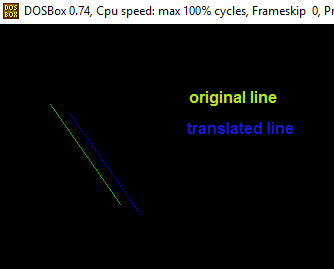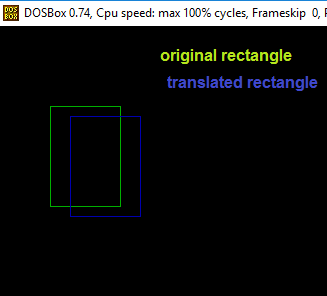# Translation of objects in computer graphics

• Difficulty Level : Easy
• Last Updated : 21 Feb, 2022

In computer graphics, we have seen how to draw some basic figures like line and circles. In this post we will discuss on basics of an important operation in computer graphics as well as 2-D geometry, which is transformation
In computer graphics, transformation of the coordinates consists of three major processes:

• Translation
• Rotation
• Scaling

In this post we will discuss about translation only.

What is translation?

A translation process moves every point a constant distance in a specified direction. It can be described as a rigid motion. A translation can also be interpreted as the addition of a constant vector to every point, or as shifting the origin of the coordinate system.
Suppose, If point (X, Y) is to be translated by amount Dx and Dy to a new location (X’, Y’) then new coordinates can be obtained by adding Dx to X and Dy to Y as:

```X' = Dx + X
Y' = Dy + Y

or P' = T + P where

P' = (X', Y'),
T = (Dx, Dy ),
P = (X, Y)```

Here, P(X, Y) is the original point. T(Dx, Dy) is the translation factor, i.e. the amount by which the point will be translated. P'(X’, Y’) is the coordinates of point P after translation.
Examples:

```Input : P[] = {5, 6}, T = {1, 1}
Output : P'[] = {6, 7}

Input : P[] = {8, 6}, T = {-1, -1}
Output : P'[] = {7, 5}```

Whenever we perform translation of any object we simply translate its each and every point. Some of basic objects along with their translation can be drawn as:

1. Point Translation P(X, Y) : Here we only translate the x and y coordinates of given point as per given translation factor dx and dy respectively.
Below is the C++ program to translate a point:

## CPP

 `// C++ program for translation``// of a single coordinate``#include``#include` `using` `namespace` `std;` `// function to translate point``void` `translatePoint ( ``int` `P[], ``int` `T[])``{``    ``/* init graph and putpixel are used for``       ``representing coordinates through graphical``       ``functions``    ``*/``    ``int` `gd = DETECT, gm, errorcode;``    ``initgraph (&gd, &gm, "c:\\tc\\bgi");``    ` `    ``cout<<"Original Coordinates :"<
1. Output:

```Original Coordinates : 5, 8
Translated Coordinates : 7, 9```
1.
2. Line Translation: The idea to translate a line is to translate both of the end points of the line by the given translation factor(dx, dy) and then draw a new line with inbuilt graphics function.
Below is the C++ implementation of above idea:

## CPP

 `// cpp program for translation``// of a single line``#include``#include` `using` `namespace` `std;` `// function to translate line``void` `translateLine ( ``int` `P[], ``int` `T[])``{``    ``/* init graph and line() are used for``       ``representing line through graphical``       ``functions``    ``*/``    ``int` `gd = DETECT, gm, errorcode;``    ``initgraph (&gd, &gm, "c:\\tc\\bgi");``    ` `    ``// drawing original line using graphics functions``    ``setcolor (2);``    ``line(P, P, P, P);` `    ``// calculating translated coordinates``    ``P = P + T;``    ``P = P + T;``    ``P = P + T;``    ``P = P + T;` `    ``// drawing translated line using graphics functions``    ``setcolor(3);``    ``line(P, P, P, P);``    ``closegraph();``}` `// driver program``int` `main()``{``    ``int` `P = {5, 8, 12, 18}; ``// coordinates of point``    ``int` `T[] = {2, 1}; ``// translation factor``    ``translateLine (P, T);``    ``return` `0;``}`
1. Output1.
2. Rectangle Translation : Here we translate the x and y coordinates of both given points A(top left ) and B(bottom right) as per given translation factor dx and dy respectively and then draw a rectangle with inbuilt graphics function

## CPP

 `// C++ program for translation``// of a rectangle``#include``#include``using` `namespace` `std;` `// function to translate rectangle``void` `translateRectangle ( ``int` `P[], ``int` `T[])``{``    ``/* init graph and rectangle() are used for``    ``representing rectangle through graphical functions */``    ``int` `gd = DETECT, gm, errorcode;``    ``initgraph (&gd, &gm, "c:\\tc\\bgi");``    ``setcolor (2);``    ``// rectangle (Xmin, Ymin, Xmax, Ymax)``    ``// original rectangle``    ``rectangle (P, P, P, P);` `    ``// calculating translated coordinates``    ``P = P + T;``    ``P = P + T;``    ``P = P + T;``    ``P = P + T;` `    ``// translated rectangle (Xmin, Ymin, Xmax, Ymax)``    ``// setcolor(3);``    ``rectangle (P, P, P, P);``    ``// closegraph();``}` `// driver program``int` `main()``{``    ``// Xmin, Ymin, Xmax, Ymax as rectangle``    ``// coordinates of top left and bottom right points``    ``int` `P = {5, 8, 12, 18};``    ``int` `T[] = {2, 1}; ``// translation factor``    ``translateRectangle (P, T);``    ``return` `0;``}`
1. Output1.

References : http://math.hws.edu/graphicsbook/.